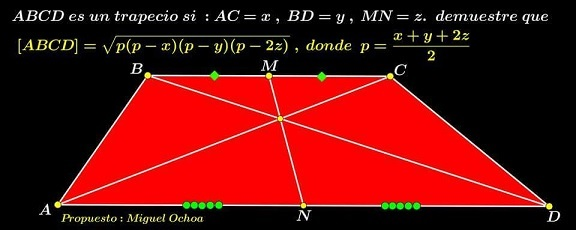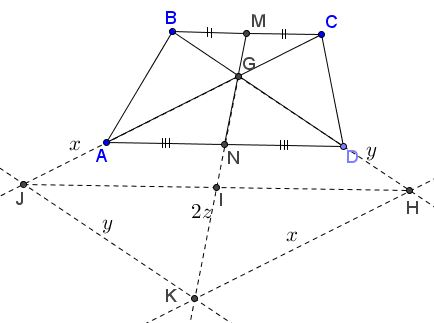# Area of Trapezoid

The following problem with solution (Proof 1 below) has been posted by Miguel Ochoa Sanchez at the CutTheKnotMath facebook page:### Problem

In trapezoid $ABCD,$ $BC\parallel AD,$ $x=AC,$ $y=BD,$ $z=MN,$ where $M$ and $N$ are the midpoints of $BC$ and $AD,$ respectively.Let $p=\displaystyle\frac{x+y+2z}{2}.$ Then, for the area $[ABCD]$ of the trapezoid, we have

$[ABCD]^{2}=p(p-x)(p-y)(p-2z).$

### Proof 1

Define $E$ the midpoint of $AB$ and $F$ that of $CD.$\displaystyle \begin{align} [BEM]+[DFN]&=\frac{1}{4}[ABC]+\frac{1}{4}[ACD]=\frac{1}{4}[ABCD], \\ {}[AEN]+[CFM]&=\frac{1}{4}[ABD]+\frac{1}{4}[BCD]=\frac{1}{4}[ABCD] \end{align}

so that

$\displaystyle [AEN]+[BEM]+[CFM]+[DFN]=\frac{1}{2}[ABCD],$

meaning $\displaystyle [EMFN]=\frac{1}{2}[ABCD].$ Hence, $\displaystyle [EMN]=\frac{1}{4}[ABCD],$ or $[ABCD]=4[EMN].$ Now, $\displaystyle EM=\frac{x}{2},$ $\displaystyle EN=\frac{y}{2},$ $MN=z.$ Apply Heron's formula to $\Delta EMN:$

\displaystyle \begin{align}[EMN]^2&=\frac{x+y+2z}{4}\cdot\frac{-x+y+2z}{4}\cdot\frac{x-y+2z}{4}\cdot\frac{x+y-2z}{4}\\ &=\frac{p}{2}\cdot\frac{p-x}{2}\cdot\frac{p-y}{2}\cdot\frac{p-2z}{2}. \end{align}

And, finally,

$\displaystyle [ABCD]^{2}=16[EMN]^{2}=16\frac{1}{16}p(p-x)(p-y)(p-2z).$

### Proof 2

Note that the three lines $AC,$ $BD,$ and $MN$ are concurrent and extend each beyond $A,$ $D,$ and $N$ by $CG,$ $BG,$ and $MG,$ respectively, to obtain points $J,$ $I,$ $H,$ as shown. Complete parallelogram with point $K.$Then $JH=AD+BC$ from which $[ABCD]=[JGH]=\frac{1}{2}[GJKH]=[GHK].$ However, in $\Delta GHK,$ $HK=x,$ $GH=y,$ and $GK=2z.$ All that remains is to apply Heron's formula in $\Delta GHK.$